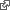#Expense Calculator

I wrote the AWK version of this calculator when I volunteered to work out the expenses for our 1981 ski trip to Jackson Hole. webpageI wasn't even sure how to approach the calculation so I just started writing facts into a file. Pretty soon I realized that if I could just sum the groups and then bring those sums into other groups then I'd pretty much have it.

I wrote AWK to do the calculation and print a report. I made it more general than need be because that was the easiest way to get it right. I finished the script and the report together.

A key notion is that there is a running sum. This is saved to or restored from a hash when a name is encountered. The first occurrence defines the name; later occurrences recall its value.

\$1 ~ /^[A-Z]+[A-Z0-9]*\$/ { if (sums[\$1] == "" || \$1 == "SUM") { # Define Symbol sums[\$1] = sum \$1 = sum sum = 0 } else { # Dereference Symbol \$1 = sums[\$1] } } # Pretty Print (\$1+0) != 0 {\$1 = sprintf("%7.2f", \$1)} {print} # Explicit Calculations \$2 == "*" {\$1 *= \$3} \$2 == "/" {\$1 /= \$3} \$2 == "DB" {\$1 = -\$1} \$2 == "CR" {\$1 = -\$1} # Implicit Summation NF == 0 {sum = 0} {sum += \$1}

My friend Jim Bessemer recognized the similarity of my approach with spreadsheet programs which I had not yet used. The common feature is calculation in the context of a document.

Years later I revived the program and recoded it in CoffeeScript as a step toward the calculation methodology for Federated Wiki.